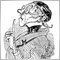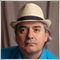# [Help] What is the Real Formula of Exponential Moving Average? Please explain it to me. I searched in internet, I found many EMA Formula. - page 2Moderator
10155

Fernando,

your approach looks quite amazing - even though it is not was I was interested in.

So I did it my self with a surprising and so does it look:

The original BB is Tomato the BBema  is Green and calculated as

//remark for all buffer the empty value is set to 0.0!
double alpha = 2.0/((double)(BandsPeriod+1));
for(i=0; i<limit; i++) {

MovingBuffer2[i]=iMA(NULL,0,BandsPeriod,BandsShift,MODE_EMA,PRICE_CLOSE,i);
diff = (Close[i] - MovingBuffer2[i]);
DeviBuffer2[i]  = DeviBuffer2[i+1]==0.0? diff*diff :  (1.0-alpha)*( DeviBuffer2[i+1] + alpha*diff*diff);
UpperBuffer2[i] = MovingBuffer2[i] + sqrt( DeviBuffer2[i] );
LowerBuffer2[i] = MovingBuffer2[i] - sqrt( DeviBuffer2[i] );
}

Am I doing something wrong?

@ Musgni: Read Chapter 9 "Exponentially-weighted mean and variance" - that's about the ema.5069

Musngi: I don't see EMA in that pdf
9. Exponentially-weighted mean and variance (Page 7)5069

Carl Schreiber: Fernando, your approach looks quite amazing - even though it is not was I was interested in.

So I did it my self with a surprising and so does it look:

The original BB is Tomato the BBema  is Green and calculated as

Am I doing something wrong?

Don't mix the iMA with the other equation. Calculate both the "Moving Average" and the "Moving Variance" with the use of the equations in the PDF, namely and I quote:

diff := x - mean
incr := alpha * diff
mean := mean + incr
variance := (1 - alpha) * (variance + diff * incr)Moderator
10155

Fernando Carreiro:

Don't mix the iMA with the other equation. Calculate both the "Moving Average" and the "Moving Variance" with the use of the equations in the PDF, namely and I quote:

diff := x - mean
incr := alpha * diff
mean := mean + incr
variance := (1 - alpha) * (variance + diff * incr)

Isn't it that  what I did?

I only used the build-in ema = mean = iMa(..., MODE_EMA, ..) - that shouldn't be the problem but (almost) the same!

mean = iMA(...);

x = Close[i];

diff = x-mean;

diff*incr = (Close[i]-iMa(..,i)*alpha*(Close[i]-iMA(..,i) ) = alpha * diff²;5069

Carl Schreiber:

Isn't it that  what I did?

I only used the build-in ema = mean = iMa(..., MODE_EMA, ..) - that shouldn't be the problem but (almost) the same!

mean = iMA(...);

x = Close[i];

diff = x-mean;

diff*incr = (Close[i]-iMa(..,i)*alpha*(Close[i]-iMA(..,i) ) = alpha * diff²;

Why use the iMA when the equation for the EMA is much faster and with less rounding errors?

Try it both ways and see for yourself to see if you get the same results.

Also, I repeat, if you are trying to make it superimpose on the original Bollinger, it will never happen - one is based on SMA and the other is based on EMA. They will never be the same.Moderator
10155

I know it will never be the same but I haven't expected such a big difference!

The normal (incremental) ema is quite similar to the sma (no interest in that as already proved!) but the variance isn't and that is surprising to me! It looks as if the smoothing is wrong.5069

Carl Schreiber:

I know it will never be the same but I haven't expected such a big difference!

The normal (incremental) ema is quite similar to the sma (no interest in that as already proved!) but the variance isn't and that is surprising to me! It looks as if the smoothing is wrong.

I will take my version of the indicator and remove all the extra stuff and leave just the standard EMA Bollinger bands and send it to you later today, so you can use it as a basis of comparison!Moderator
10155

Fernando Carreiro:

I will take my version of the indicator and remove all the extra stuff and leave just the standard EMA Bollinger bands and send it to you later today, so you can use it as a basis of comparison!

Fernando,

Don't worry about this!! I don't want to blame your indicator (in contrary) - I just wanted to get a feeling about the variance calculated acc. to the article and I am very much surprised about the difference which is a lot bigger that the difference between ema and sma.5069

Carl Schreiber:

Fernando,

Don't worry about this!! I don't want to blame your indicator (in contrary) - I just wanted to get a feeling about the variance calculated acc. to the article and I am very much surprised about the difference which is a lot bigger that the difference between ema and sma.

As promised, here is an EMA with Bollinger-type bands which you can then compare to the standard BB. It may have extra unnecessary or bloated code because I just whittled it down from a more complex indicator.
Files:Moderator
10155

I have found my problem:

//remark: for all buffers the empty value is set to 0.0!
double alpha = 2.0/((double)(BandsPeriod+1));
i = limit;
while(i-->0) {  //  sorry I took parts of someone else code and didn't check that i has to go from the past to present!
MovingBuffer2[i]=iMA(NULL,0,BandsPeriod,BandsShift,MODE_EMA,PRICE_CLOSE,i);
diff = (Close[i] - MovingBuffer2[i]);
DeviBuffer2[i] = DeviBuffer2[i+1]==0.0? diff*diff : (1.0-alpha)*( DeviBuffer2[i+1] + alpha*diff*diff);
UpperBuffer2[i] = MovingBuffer2[i] + sqrt( DeviBuffer2[i] );
LowerBuffer2[i] = MovingBuffer2[i] - sqrt( DeviBuffer2[i] );
}

Now it looks like:

Green are the EMA-Bands Tomato the original BB.